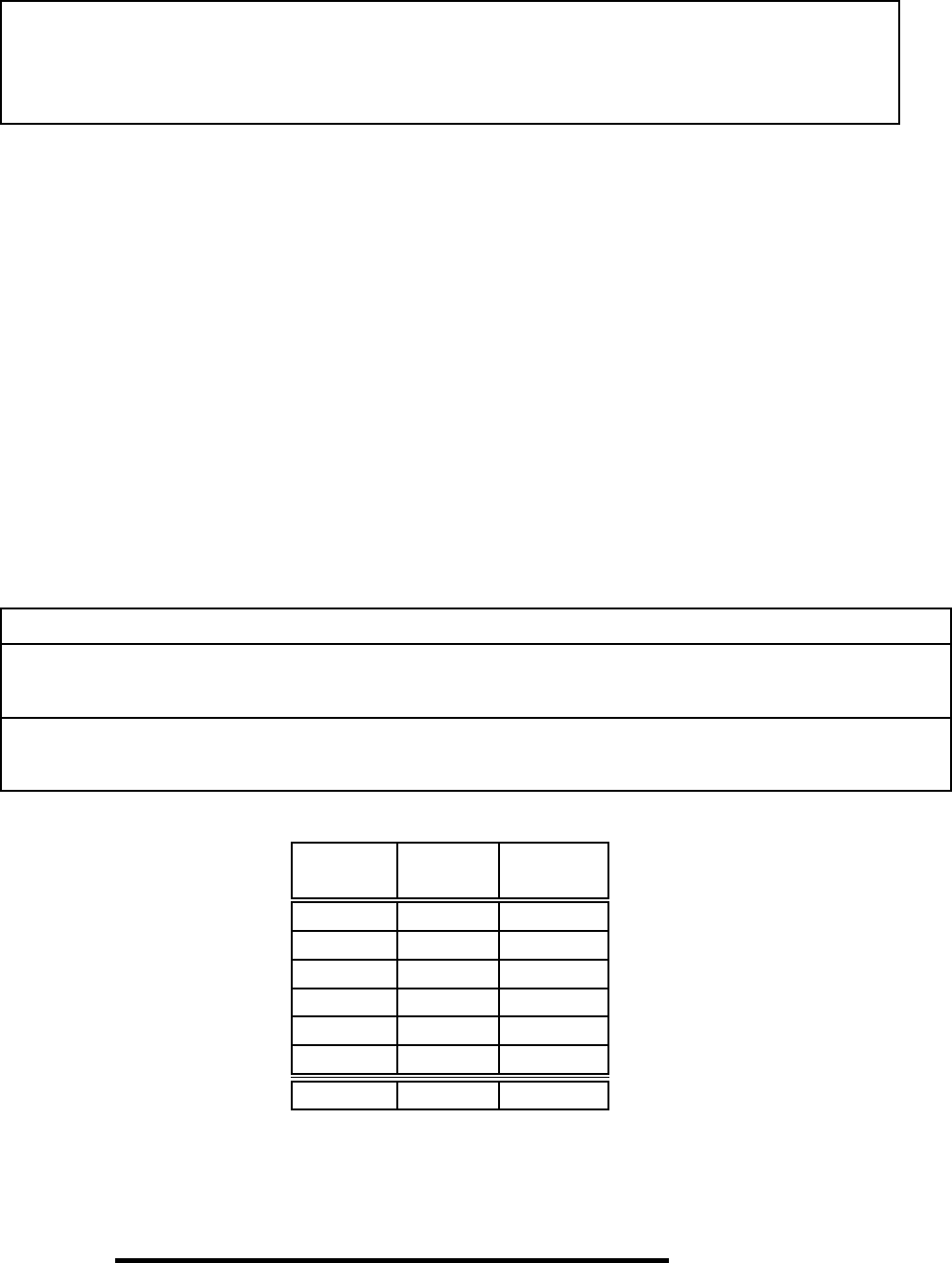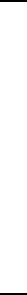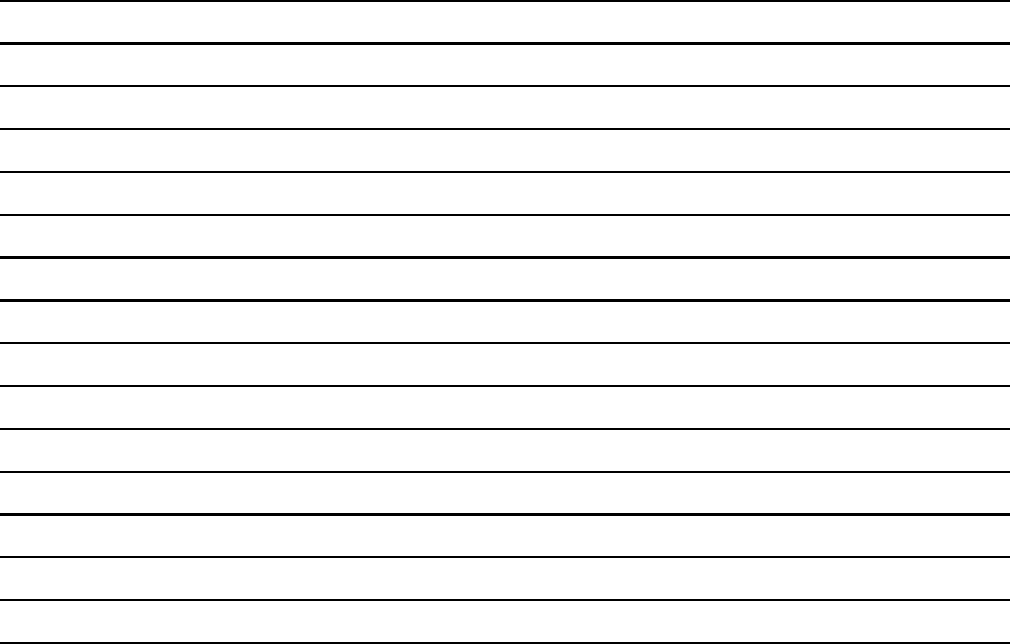Class Notes (1,100,000)
US (490,000)
WUSTL (3,000)
E81 CSE (40)
coles (4)
Lecture 6

# Computer Science And Engineering CSE 232 Lecture Notes - Lecture 6: Pseudocode, Binary Search Algorithm, Linked ListExam

Department
Computer Science And Engineering
Course Code
Computer Science And Engineering CSE 232
Professor
coles
Lecture
6

This preview shows pages 1-3. to view the full 10 pages of the document.CSE 247 Data Structures and Algorithms Spring 2017
Exam I
Given: 1 March 2017 Due: End of session
This exam is closed-book, closed-notes. No electronic devices or resources of any kind are
allowed. The exception is the “cheat sheet” as described on our course web pages on which you
may have notes to consult during the exam.
Your wo rk mus t b e legible. Work that is dicult to read will receive no credit. Do not dwell over
punctuation or exact syntax in code; however, be sure to indent your code to show its structure.
You mu st sign the pledge b elow for you r exam to count. Any ch eating will cause the students
involved to receive an F for this course. Other action may be taken.
Your work must b e completed on these pages. Th ere is blank space on some pages of the exam
if you need it. Don’t spend too much time staying stuck on a question: move on and return to the
question later.
You mu st ﬁll in your identifying information correctly. Whenyoureachthispointinthe
instructions, please give the professor or TA a meaningful glance.
Print clearly the following information:
Name (print clearly):
Student 6-digit ID (print really clearly):
Number Points Points
120
210
320
410
520
620
Total 100
Pledge: On my honor, I have neither given nor received any unauthorized aid on this exam.
Signed:
(Be sure you ﬁlled in your information in the box above!)

Only pages 1-3 are available for preview. Some parts have been intentionally blurred.CSE 247 (Exam I) –2– Due by End of session
1. (20 points)
(a) (5 points) log n=O(g(n)) for which of the following deﬁnitions of g(n)?
Circle all that apply:
g(n)=1
g(n) = log n+247
g(n)=n
g(n)=nlog n
g(n)=n2log n
None of the above
(b) (5 points) log n=(g(n)) for which of the following deﬁnitions of g(n)?
Circle all that apply:
g(n)=1
g(n) = log n+247
g(n)=n
g(n)=nlog n
g(n)=n2log n
None of the above
Read the following questions carefully: they are phrased dierent from
the above.
(c) (5 points) f(n)=Θ(n) for which of the following deﬁnitions of f(n)?
Circle all that apply:
f(n)=0
f(n)=247
f(n)= n
247
f(n)=247n
f(n)=247n+131
None of the above
(d) (5 points) f(n)=Θ(0) for which of the following deﬁnitions of f(n)?
Circle all that apply:
f(n)=0
f(n)=247
f(n)= n
247
f(n)=247n
f(n)=247n+131
None of the above

Only pages 1-3 are available for preview. Some parts have been intentionally blurred.CSE 247 (Exam I) –3– Due by End of session
2. (10 points) Recall that f(n)=O(g(n)) if
c>0n0>0|nn0f(n)c·g(n)
Prove that 2n=O(n!).
To attempt full credit, you do this by induction on n, starting at n=0. You
must use the induction hypothesis
P(n):2
n=O(n!)
You must explicitly show P(0) and then show P(k)P(k+1)
Or, for attempt at most half credit, you do a direct proof based on analysis of 2n
and n!.
(a) Circle only one of the above two methods, indicating which proof technique you
are going to use.
(b) Using the notation above, write your proof below. Where needed, state appropri-
ate values for cand n0.